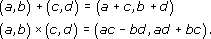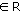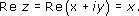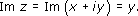Complex numbers

This free course is available to start right now. Review the full course description and key learning outcomes and create an account and enrol if you want a free statement of participation.

Free course

# 2.3 Section summary

In this section we have seen that the complex number system is the set R × R together with the operations + and × defined byFrom this, one can justify the performance of ordinary algebraic operations on expressions of the form a + ib where a, band i is an undefined symbol with the property that i2 is replaced by −1.

In the complex number system, any quadratic expression can be factorised into two linear factors.

The real part of a complex number z = x + iy is x and we writeThe imaginary part of a complex number z = x + iy is y and we writeTwo complex numbers are equal if and only if their real parts are equal and their imaginary parts are equal.

The complex number system obeys the usual algebraic laws of manipulation of numbers but the inequality relation, <, does not carry over from the real numbers to the complex numbers.

## Examples

(i) (3 + i) (4 −i)  = 12−i2 + 4i−3i  = 13  + i.

(ii) (6 + 7i)−(3−i) = 3 + 8i.

(iii) (x + iy)(xiy) = x2 + y2.

(iv) Re (6−4i)  = 6 , and Im (6−4i) = −4.

(v) xy−(x2 + y2)i = 1 = (x,yR).

then, by equating real and imaginary parts, we have xy =  1 and x2 +  y2 =  3

Thus the point (x, y) lies on the hyperbola xy = 1 and on the circle x2 + y2 = 3 and can take four possible values, corresponding to the points of intersection of the hyperbola and the circle.

M332_1

### Take your learning further

Making the decision to study can be a big step, which is why you'll want a trusted University. The Open University has 50 years’ experience delivering flexible learning and 170,000 students are studying with us right now. Take a look at all Open University courses.

If you are new to university level study, find out more about the types of qualifications we offer, including our entry level Access courses and Certificates.

Not ready for University study then browse over 900 free courses on OpenLearn and sign up to our newsletter to hear about new free courses as they are released.

Every year, thousands of students decide to study with The Open University. With over 120 qualifications, we’ve got the right course for you.

Request an Open University prospectus